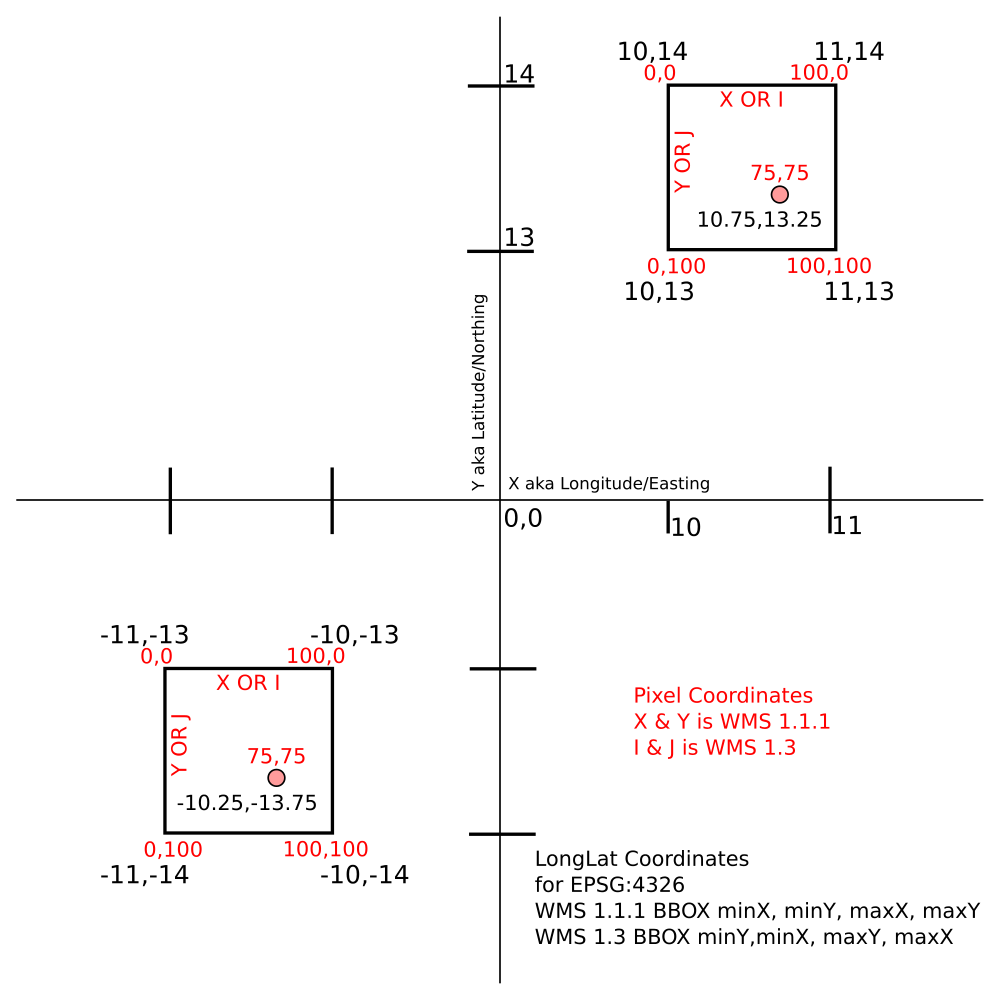The basic issue when using OGC web services is there’s no simple way to ask for the value of a layer at a specific Latitude & Longitude. WMS and WMTS require that you specify bounding boxes(BBOX) or tiles, service version, pixel coordinates and whole bunch of other stuff. WFS requires that the layer be a Vector layer, I have mostly Raster layers. What happens if you just want a raster cell value for one point. I suppose you could query that pixel from a WCS but you’d have use knowledge of the size of the original data pixels to make a BBOX

Example for 10.75,13.25

``````http://example.com/maps?MAP=map1&QUERY\_LAYERS=h11&LAYERS=h11&SERVICE=WMS&VERSION=1.1.1&REQUEST=GetFeatureInfo&STYLES=default&SRS=EPSG:4326&FEATURE\_COUNT=1&INFO\_FORMAT=text/html?BBOX=10,13,11,14&WIDTH=100&HEIGHT=100&X=75&Y=75
``````

A lot of the required parameters really don’t change for a given use case. In this project, I’m always querying in Lat Lon WGS84 and I’m not necessarily loading a map that corresponds. So I came up with a way to shorten out all the repetitive stuff and simplify the components for the request without having to abandon using the WMS server I already had serving the data.

With that knowledge and Apache Rewrite you can simply this quite a bit.

``````<IfModule rewrite_module>
RewriteEngine  on
RewriteRule "^/api/maps/([^/]*)/layers/([^/]*)\$" "/maps?MAP=\$1&QUERY_LAYERS=\$2&LAYERS=\$2&SERVICE=WMS&VERSION=1.1.1&REQUEST=GetFeatureInfo&STYLES=default&SRS=EPSG:4326&FEATURE_COUNT=1&INFO_FORMAT=text/html" [PT,QSA]
</IfModule>
``````

The end result is now you can write a more sensible url in REST style (almost)

Pattern

`maps/\<mapfile\>/layers/\<layername\>?BBOX=minX,minY,maxX,maxY&&WIDTH=100&HEIGHT=100&X=75&Y=75`

Example

``````http://example.com/api/maps/map1/layers/h11?BBOX=10,13,11,14&WIDTH=100&HEIGHT=100&X=75&Y=75
``````

The trick here, is make a 1x1 degree box based on rounding your coordinates to the nearest integer. Then using the remainder to get the fractional distance out of the 100x100 pixels in the request. You can also use 1000x1000 which WMS servers will allow to get 1 extra decimal place added accuracy.

The big Gotchas

• Pixel space 0,0 is upper left corner (positive numbers go down)
• WMS 1.3 for EPSG:4326 swaps the Lat Lon ordering in the BBOX
• Negative coordinates require a little extra handling.

See diagram below.Note: For WMS 1.3 it’s CRS not SRS, and I & J not X & Y

And now an example of how to build the request url in Javascript. If you’re WMS service is set to return json or geojson or html that should be all you need.

``````function getInfoUrl(lonlat) {

//retrieve the name of actively selected layer you want to query
var layername = \$("#variableSelect :selected").val();

var lon = lonlat;
var lat = lonlat;

var minx = Math.floor(lon);
var maxx = minx+1;
var miny = Math.floor(lat);
var maxy = miny+1;

var bbox = "BBOX="+minx+","+miny+","+maxx+","+maxy;
var wh = "WIDTH=100&HEIGHT=100";
//Pixel within bbox at given width and height
if (lon < 0) {
var x = Math.round(100+((lon % 1)*100));
} else {
var x = Math.round((lon % 1)*100);
}
if (lat < 0) {
var y= Math.round(Math.abs((lat % 1)*100));
} else {
var y = Math.round(100-((lat % 1)*100));
}

var baseurl = "http://test2.biogeo.ucdavis.edu/api/maps/dhsmap/layers/"
var fullurl = baseurl+layername+"?"+bbox+"&"+wh+"&X="+x+"&Y="+y;
//console.log(lonlat);
//console.log(fullurl);
return(fullurl);

}
``````

It would be awesome to eliminate the BBOX part and the pixel space coordinates so all you need is Lat & Long. But so far it looks like one needs to write a small web application to do that.